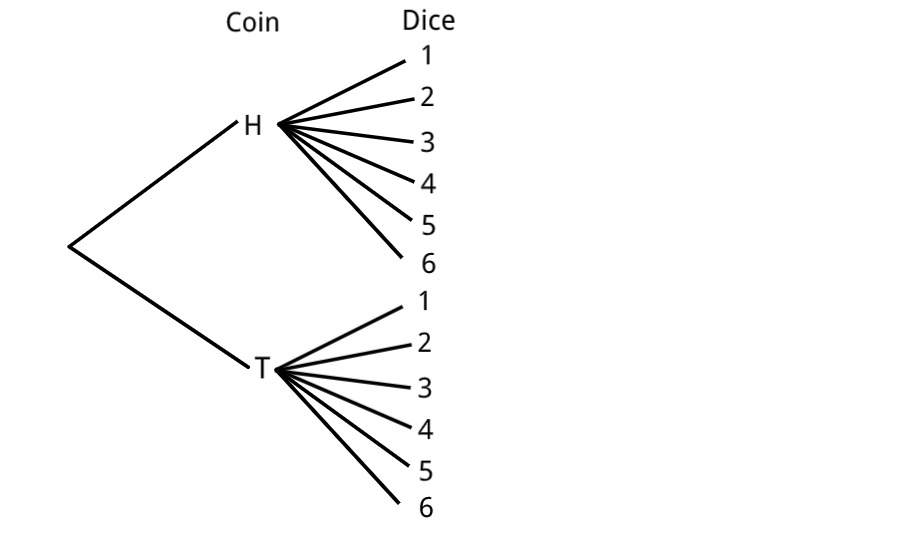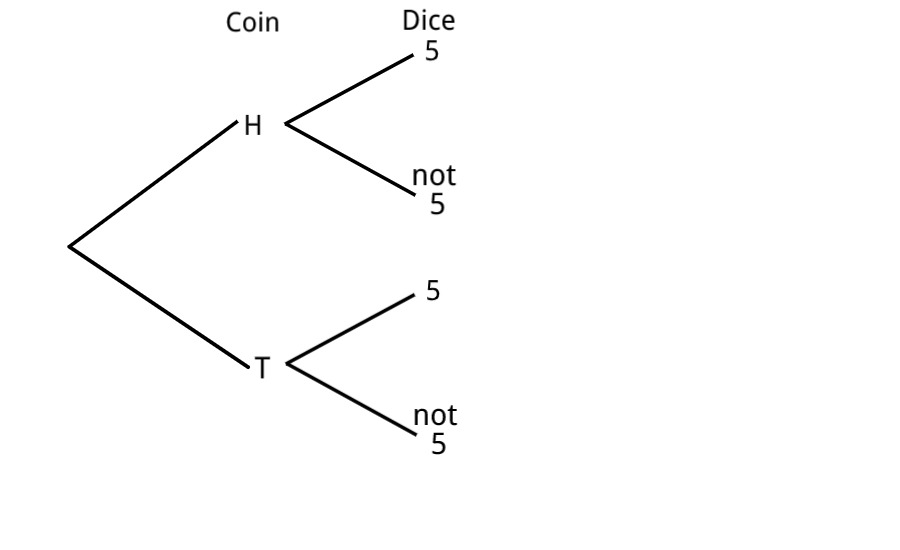# 3.1 Introducing the Product Rule

Last updated: February 25th, 2020

# Introducing the Product Rule¶

In this section we are going to introduce some rules that will allow us to calculate each time more complex probabilities.

Imagine the following experiment: we flip a coin and then throw a dice and write down both results. We want the probability of getting Heads and a $5$.

In this case, since this is a "small" example, we can solve it as we already know. So let's first do that, and then I'll show you another way of doing it that will be useful when we start having "bigger" examples.

Notice that both the coin and the dice are fair, so our experiment has equally likely results. And $\Omega=\{H1, H2, H3, H4, H5, H6, T1, T2, T3, T4, T5, T6\}$.

Thus, $P(H5)=\dfrac{1}{|\Omega|}=\dfrac{1}{12}$ because getting Heads and $5$ is one individual outcome out of the $12$.

Now, most of the times, $\Omega$ will be really big, so it won't be a good idea to list each and every element.

What we'll do instead is what we call a tree diagram:Notice how the first branching has two options giving us a probability of $\dfrac{1}{2}$, and the second branching has six options giving us a probability of $\dfrac{1}{6}$.

Hence, to get the $\dfrac{1}{12}$ that we need, we can multiply those two individual probabilities: $P(H and \ 5)=P(H)\times P(5)=\dfrac{1}{2}\times\dfrac{1}{6}=\dfrac{1}{12}$.

Still, it's already a bit tedious to have those six branches, so instead we are going to focus on the outcome we are interested in:But again, we get that the probability along the branch we want is the product of the two individual branches.

In general, when we have two (or more) outcomes/events and we want the probability of them happening at the same time, we are going to multiply the two individual probabilities.

Of course, this is going to be called the Product Rule.

In terms of the Tree Diagram, we can say that the probability across one branch is the product of the probabilities.

One final and important remark: The Product Rule has certain conditions that need to be met in order to use it, but we are going to dig deeper into that in future videos.